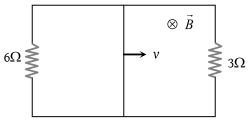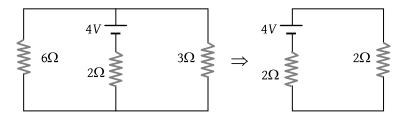# NEET Questions Solved

A rectangular loop with a sliding connector of length l = 1.0 m is situated in a uniform magnetic field B = 2T perpendicular to the plane of loop. Resistance of connector is r = 2Ω. Two resistance of 6Ω and 3Ω are connected as shown in figure. The external force required to keep the connector moving with a constant velocity v = 2m/s is(1) 6 N

(2) 4 N

(3) 2 N

(4) 1 N

Concept Videos :-

#6 | Motional EMF (Induced Electric Field)
#7 | Problem Set1: Motional EM
#8 | Problem Set2: Motional EM
#9 | Problem Set3: Motional EMF
#10 | Problem Set4: Motional EMF
#11 | Equivalent Circuit Diagrams

Concept Questions :-

Motional emf

(3) Motional emf $e=Bvl⇒e=2×2×1=4\text{\hspace{0.17em}}V$

This acts as a cell of emf E = 4V and internal resistance r = 2Ω.

This simple circuit can be drawn as followsCurrent through the connector $i=\frac{4}{2+2}=1\text{\hspace{0.17em}}A$

∴ magnetic force on connector ${F}_{m}=Bil=2×1×1=2\text{\hspace{0.17em}}N$  (Towards left)

Difficulty Level:

• 10%
• 35%
• 51%
• 4%
Crack NEET with Online Course - Free Trial (Offer Valid Till September 19, 2019)